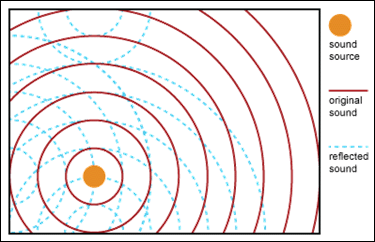# Doubling of Distance Noise Reduction

eNoise Control presents the following information regarding doubling of distance. This information can also be found in the OSHA technical manual, Section III, Chapter 5.

## Sound Pressure Level Decreases 6dB – with the Doubling of Distance from the Sound Source

A free field is a region in which there are no reflected sound waves. In a free field, sound radiates into space from a source uniformly in all directions. The sound pressure produced by the source is the same in every direction at equal distances from the point source. As a principle of physics, the sound pressure level decreases 6dB, on a Z-weighted (i.e., unweighted) scale, each time the distance from the point is doubled. This is a common way of expressing the inverse-square law in acoustics and is shown in Figure 4.

Figure 4. Sound Pressure Levels in a Free FieldIf a point source in a free field produces a sound pressure level of 90 dB at a distance of 1 meter, the sound pressure level is 84 dB at 2 meters, 78 dB at 4 meters, and so forth. This principle holds true regardless of the units used to measure distance.

Free field conditions are necessary for certain tests, where outdoor measurements are often impractical. Some tests need to be performed in special rooms called free field or anechoic (echo-free) chambers, which have sound absorbing walls, floors, and ceilings that reflect practically no sound.

In spaces defined by walls, however, sound fields are more complex. When sound-reflecting objects such as walls or machinery are introduced into the sound field, the wave picture changes completely. Sound reverberates, reflecting back into the room rather than continuing to spread away from the source. Mose industrial operations and many construction tasks occur under these conditions. Figure 5 diagrams sound radiating from a sound source and shows how reflected sound (dashed lines) complicates the situation.

Figure 5. Original and Reflected Sound WavesThe net result is a change in the intensity of the sound. The sound pressure does not decrease as rapidly as it would in a free field. In other words, it decreases by less than 6 dB each time the distance from the sound source doubles.

Far from the noise source – unless the boundaries are very absorbing – the reflected sound dominates. This region is called the reverberant field. If the sound pressure levels in a reverberant field are uniform throughout the room, and the sound waves travel in all directions with equal probability, the sound is said to be diffuse.

In actual practice, however, perfectly free fields and reverberant fields rarely exist – most sound fields are something in between.